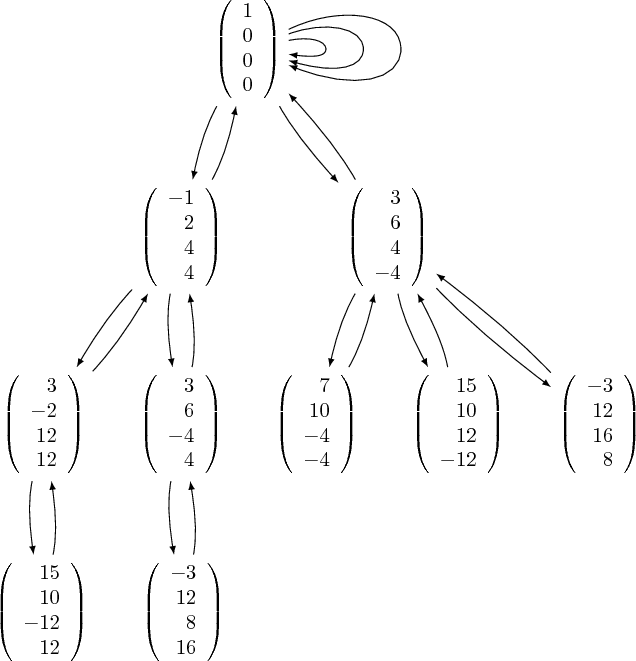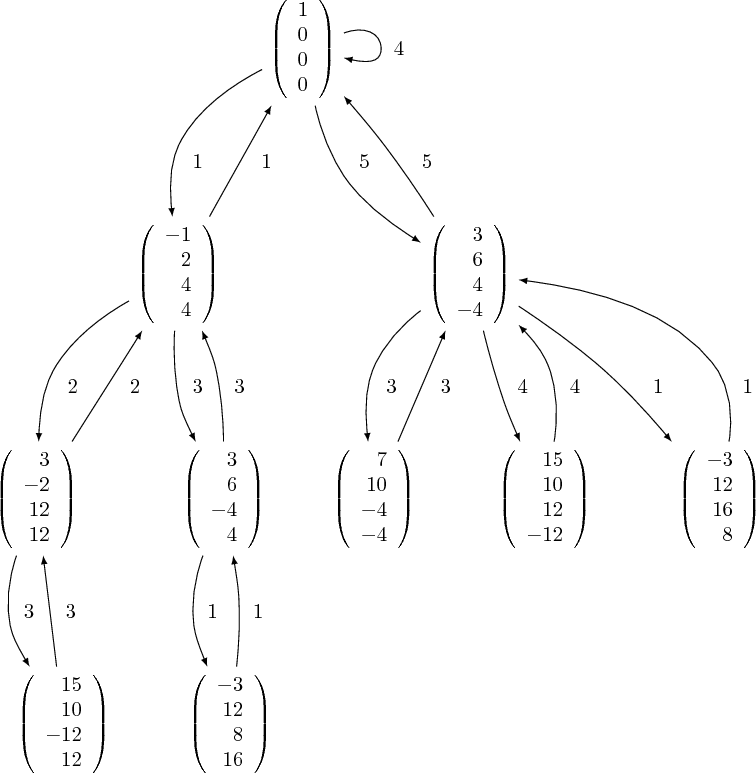# tree of vectors

This is a more complicated version of apply functions iteratively

I have a starting "seed" vector, say e1 = matrix(4,1,(1,0,0,0)) Next, I have a collection of 4x4 matrices

thin_group = [T1,T2,T3,T4,T5]

where

$T1 = \left(\begin{array}{rrrr} -1 & 0 & 0 & 0 \\ 2 & 1 & 0 & 0 \\ 4 & 0 & 1 & 0 \\ 4 & 0 & 0 & 1 \end{array}\right), T2 = \left(\begin{array}{rrrr} 1 & 2 & 0 & 0 \\ 0 & -1 & 0 & 0 \\ 0 & 4 & 1 & 0 \\ 0 & 4 & 0 & 1 \end{array}\right), T3 = \left(\begin{array}{rrrr} 1 & 0 & 1 & 0 \\ 0 & 1 & 1 & 0 \\ 0 & 0 & -1 & 0 \\ 0 & 0 & 0 & 1 \end{array}\right), T4 = \left(\begin{array}{rrrr} 1 & 6 & 0 & 6 \\ 0 & 3 & 0 & 2 \\ 0 & 4 & 1 & 4 \\ 0 & -4 & 0 & -3 \end{array}\right), T5 = \left(\begin{array}{rrrr} 3 & 0 & 0 & 2 \\ 6 & 1 & 0 & 6 \\ 4 & 0 & 1 & 4 \\ -4 & 0 & 0 & -3 \end{array}\right)$

These matrices either fix e1 or send to a different vector. I have a "height" function, which just measures how large the vector entries are getting.

def h(X): return max(abs(X[0,0],abs(X[1,0]), abs(X[2,0]),abs(X[3,0]))

I want to develop the following procedure:

hmax = 50 Begin applying T1,...,T5 to e1. As soon as I get a different vector (T1*e1 = (-1,2,4,4)), measure the height of that vector. If the height is below hmax (h(-1,2,4,4) = 4 < hmax = 50), store that vector somewhere. Now apply another matrix from thin_group to (-1,2,4,4), (but not T1 again). If the new vector has a height below hmax, store it, and apply another matrix from thin_group, just not the previous one. Continue this process until we reach a vector with a height larger than hmax. Once this happens, go back to the previous vector and apply a different matrix from thin_group i.e. go up a different branch of the tree. Go up this branch until hmax is exceeded, then go up a different branch, etc.. Eventually, this process will generate all thin_group images of e1 with hmax < 50. (this is probably about 50,000 vectors)

This procedure should "remember" the vectors that were counted/stored, so I'm thinking that I may be able to index each vector in the tree with a sequence (a1,a2,a3,...,an) where aj=1,2,3,4,5 to denote which matrix T1,...,T5 we applied previously.

Any/all ideas or partial solutions greatly appreciated. Thanks!

edit retag close merge delete

Sort by » oldest newest most voted

I think you want to use RecursivelyEnumeratedSet in sage which consist in applying iteratively a successor function to a set of initial seeds:

sage: v = matrix(4,1,(1,0,0,0))
sage: v.set_immutable()
sage: seed = [v]
sage: T1 = matrix( ZZ, 4, [ -1,0,0,0,  2, 1,0,0,  4,0, 1,0,  4, 0,0, 1 ] )
sage: T2 = matrix( ZZ, 4, [  1,2,0,0,  0,-1,0,0,  0,4, 1,0,  0, 4,0, 1 ] )
sage: T3 = matrix( ZZ, 4, [  1,0,1,0,  0, 1,1,0,  0,0,-1,0,  0, 0,0, 1 ] )
sage: T4 = matrix( ZZ, 4, [  1,6,0,6,  0, 3,0,2,  0,4, 1,4,  0,-4,0,-3 ] )
sage: T5 = matrix( ZZ, 4, [  3,0,0,2,  6, 1,0,6,  4,0, 1,4, -4, 0,0,-3 ] )
sage: thin_group = [T1, T2, T3, T4, T5]
sage: def succ(u):
....:     ans = []
....:     for m in thin_group:
....:         m_u = m*u
....:         m_u.set_immutable()
....:         ans.append(m_u)
....:     return ans
....:
sage: R = RecursivelyEnumeratedSet(seed, succ, max_depth=4)
sage: R
A recursively enumerated set (breadth first search) with max_depth=4


To get a finite set, you may want to use max_depth as above or hmax as you suggest:

sage: def the_rec_enum_set(hmax):
....:     def succ(u):
....:         ans = []
....:         for m in thin_group:
....:             m_u = m*u
....:             m_u.set_immutable()
....:             if max(map(abs, m_u)) < hmax:
....:                 ans.append(m_u)
....:         return ans
....:     R = RecursivelyEnumeratedSet(seed, succ)
....:     return R
....:
sage: the_rec_enum_set(20)
A recursively enumerated set (breadth first search)


The recursively enumerated set can be enumerated in different maner (look at the documentation). What I like the most is to create the generated graph:

sage: G = the_rec_enum_set(20).to_digraph(); G
Looped multi-digraph on 10 vertices
sage: G = the_rec_enum_set(50).to_digraph(); G
Looped multi-digraph on 37 vertices
sage: G = the_rec_enum_set(500).to_digraph(); G
Looped multi-digraph on 775 vertices


The above numbers confirm what dan_fulea obtain.

Then, you may want to use my optional package to visualize it (with dot2tex and graphviz installed):

sage -pip install slabbe
sage -i dot2tex


and then:

sage: from slabbe import TikzPicture
sage: G = the_rec_enum_set(20).to_digraph()
sage: tikz = TikzPicture.from_graph(G)
sage: tikz.pdf()        # or tikz.png() or tikz.svg()Looking at the picture, your structure looks to be symmetric (y is a successor of x if and only if x is a successor of y). If so, use

sage: R = RecursivelyEnumeratedSet(seed, succ, structure='symmetric')


which allows to enumerate all elements without keeping all of them in memory. Even better, if your structure is really a tree or a forest (that is if you are able to avoid backward arrows and any loop in the successor function), then use

sage: R = RecursivelyEnumeratedSet(seed, succ, structure='forest')


which will allow you to enumerate the vectors in parallel using fast map reduce algorithms written by Florent Hivert without keeping the elements in memory. In any case, first consult the documentation I have written:

sage: RecursivelyEnumeratedSet?


One information that is missing in the graph is the label of the edges. One way to solve this is to momentarily hide the label information inside the arrival vertex. Once, the graph is made, we put back the information into the edge. I usually do like this:

sage: seed = [(v,None)]
sage: def the_rec_enum_set(hmax):
....:     def succ(node):
....:         u,label = node
....:         ans = []
....:         for i,m in zip([1,2,3,4,5], thin_group):
....:             m_u = m*u
....:             m_u.set_immutable()
....:             if max(map(abs, m_u)) < hmax:
....:                 ans.append((m_u,i))
....:         return ans
....:     R = RecursivelyEnumeratedSet(seed, succ)
....:     return R
....:
sage: R = the_rec_enum_set(20)
sage: G = R.to_digraph()
sage: G
Looped multi-digraph on 22 vertices
sage: from slabbe.graph import digraph_move_label_to_edge
sage: GG = digraph_move_label_to_edge(G)
sage: GG
Looped digraph on 10 vertices
sage: TikzPicture.from_graph(GG).pdf()I intend to move this feature directly in Sage once I have time. Tell me if you have any other comment, I intend to improve this module in a near future.

more

Very nice code, thank you. Can I ask, in line 4, why did you use random matrices?

That was my first attempt. I edited my answer later on using your matrices instead of random ones, but I forgot at some point to edit that line. It should be ok now.

Ok got it. In some cases, my thin_group may have loops and in other cases not. The visualization is very nice!

On the last line tikz.pdf(), I don't get any output. I have dot2tex, graphviz, and slabbe installed. Any idea why this may be so?

Maybe it depends on the way you use Sage? On the terminal, it works for me. Also in the ipython notebook, you may try:

tikz = TikzPicture.from_graph(G)
path_to_file = tikz.png()
from ipython.display import Image
Image(path_to_file)


My generating statistics differs, so i hope i understood the question well. Here is the quick code:

T1 = matrix( ZZ, 4, [ -1,0,0,0,  2, 1,0,0,  4,0, 1,0,  4, 0,0, 1 ] )
T2 = matrix( ZZ, 4, [  1,2,0,0,  0,-1,0,0,  0,4, 1,0,  0, 4,0, 1 ] )
T3 = matrix( ZZ, 4, [  1,0,1,0,  0, 1,1,0,  0,0,-1,0,  0, 0,0, 1 ] )
T4 = matrix( ZZ, 4, [  1,6,0,6,  0, 3,0,2,  0,4, 1,4,  0,-4,0,-3 ] )
T5 = matrix( ZZ, 4, [  3,0,0,2,  6, 1,0,6,  4,0, 1,4, -4, 0,0,-3 ] )

T_List = ( T1, T2, T3, T4, T5 )
v = vector( ZZ, 4, [ 1,0,0,0 ] )
v = v.column()

def ht( w ):
return max( [ abs(entry) for entry in w ] )

def fillList( T_List, vector_List, height ):
v_List = vector_List[:]    # and we will append
v_New  = vector_List[:]    # use them to generate new vectors, this is the "last wave"

count = 0    # give me some small control...
while v_New and count < 100 :
count += 1    # this will protect me against the usual programming errors
w_New = []    # and we will append, this is the "new wave"
for v in v_New:
for T in T_List:
w = T*v
if w not in v_List and ht(w) < height:
w_New.append( w )
v_List += w_New
L = len( w_New )
print "%s new entr%s added..." % ( L, 'y was' if L == 1 else 'ies were' )
v_New = w_New[:]

print "A list with %s vectors was generated in %s steps" % ( len( v_List ), count )
return v_List

for height in ( 20, 50, 500 ):
print 60*'-'
print "Generation for height %s" % height
v_List = fillList( T_List, [ v, ], height )


Results:

------------------------------------------------------------
Generation for height 20
A list with 10 vectors was generated in 4 steps
------------------------------------------------------------
Generation for height 50
A list with 37 vectors was generated in 7 steps
------------------------------------------------------------
Generation for height 500
A list with 775 vectors was generated in 22 steps
sage:


As i said, possibly i am missing something... (Have to post and catch that train.)

more

Dan: very nice code. I'm still working on checking it with what I have here, but it appears to agree with my hand calculation for vectors of ht < 20. Thank you